# Hardest colleges to get into in Tennessee

Top 10 colleges in Tennessee with the lowest acceptance rates
What are the hardest colleges to get into in Tennessee? We've got you covered. We've compiled a national college database and have created a list of the hardest universities to get into in Tennessee below. These are the hardest 4 year colleges to get into in Tennessee, and sometimes some of the hardest colleges to get into in the US. You could even say these are the best colleges in Tennessee. We also include each college's average SAT and ACT scores so that you can see where you're most competitive. Read on to find out more.

## Vanderbilt University acceptance rate

Vanderbilt University acceptance rate is 9.6%.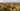The average SAT score for Vanderbilt University is 1505.

• SAT composite: 1505
• SAT math: 775

The average ACT score for Vanderbilt University is 34.

## Rhodes College acceptance rate

Rhodes College acceptance rate is 44.6%.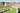The average SAT score for Rhodes College is 1330.

• SAT composite: 1330
• SAT math: 660

The average ACT score for Rhodes College is 29.

## Cumberland University acceptance rate

Cumberland University acceptance rate is 46.4%.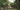The average SAT score for Cumberland University is 1050.

• SAT composite: 1050
• SAT math: 520

The average ACT score for Cumberland University is 22.

## Tennessee State University acceptance rate

Tennessee State University acceptance rate is 51.8%.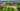The average SAT score for Tennessee State University is 980.

• SAT composite: 980
• SAT math: 490

The average ACT score for Tennessee State University is 18.

## Bryan College acceptance rate

Bryan College acceptance rate is 54.3%.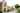The average SAT score for Bryan College is 1100.

• SAT composite: 1100
• SAT math: 530

The average ACT score for Bryan College is 23.

## Christian Brothers University acceptance rate

Christian Brothers University acceptance rate is 56%.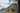The average SAT score for Christian Brothers University is 1180.

• SAT composite: 1180
• SAT math: 590

The average ACT score for Christian Brothers University is 24.

## Tennessee Wesleyan College acceptance rate

Tennessee Wesleyan College acceptance rate is 57.7%.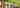The average SAT score for Tennessee Wesleyan College is 1030.

• SAT composite: 1030
• SAT math: 510

The average ACT score for Tennessee Wesleyan College is 22.

## King University acceptance rate

King University acceptance rate is 58%.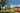The average SAT score for King University is 1050.

• SAT composite: 1050
• SAT math: 510

The average ACT score for King University is 20.

## Lincoln Memorial University acceptance rate

Lincoln Memorial University acceptance rate is 59.7%.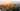The average SAT score for Lincoln Memorial University is 1110.

• SAT composite: 1110
• SAT math: 550

The average ACT score for Lincoln Memorial University is 21.

## Union University acceptance rate

Union University acceptance rate is 60%.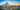The average SAT score for Union University is 1178.

• SAT composite: 1178
• SAT math: 580